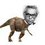# RMO Inequality Practice

If $a,b,c \in \mathbb{R}^{+}$ , such that $abc=1$ , then prove that $\displaystyle\sum_{cyc} \dfrac{a}{b} \geq \sum_{cyc} a$

Instruction: Those who have complete solution ready , please don't post it immediately.Post it minimum after 3 days of posting this note or give a small hint but not the solution.Note by Nihar Mahajan
5 years, 10 months ago

This discussion board is a place to discuss our Daily Challenges and the math and science related to those challenges. Explanations are more than just a solution — they should explain the steps and thinking strategies that you used to obtain the solution. Comments should further the discussion of math and science.

When posting on Brilliant:

• Use the emojis to react to an explanation, whether you're congratulating a job well done , or just really confused .
• Ask specific questions about the challenge or the steps in somebody's explanation. Well-posed questions can add a lot to the discussion, but posting "I don't understand!" doesn't help anyone.
• Try to contribute something new to the discussion, whether it is an extension, generalization or other idea related to the challenge.

MarkdownAppears as
*italics* or _italics_ italics
**bold** or __bold__ bold
- bulleted- list
• bulleted
• list
1. numbered2. list
1. numbered
2. list
Note: you must add a full line of space before and after lists for them to show up correctly
paragraph 1paragraph 2

paragraph 1

paragraph 2

[example link](https://brilliant.org)example link
> This is a quote
This is a quote
    # I indented these lines
# 4 spaces, and now they show
# up as a code block.

print "hello world"
# I indented these lines
# 4 spaces, and now they show
# up as a code block.

print "hello world"
MathAppears as
Remember to wrap math in $$ ... $$ or $ ... $ to ensure proper formatting.
2 \times 3 $2 \times 3$
2^{34} $2^{34}$
a_{i-1} $a_{i-1}$
\frac{2}{3} $\frac{2}{3}$
\sqrt{2} $\sqrt{2}$
\sum_{i=1}^3 $\sum_{i=1}^3$
\sin \theta $\sin \theta$
\boxed{123} $\boxed{123}$

Sort by:

Multiplying by $abc$ and making the right side homogenous, it suffices to prove: $a^2c + b^2a + c^2b \geq (abc)^{\frac{2}{3}}(a+b+c) = a^{\frac{5}{3}}b^{\frac{2}{3}}c^{\frac{2}{3}} + a^{\frac{2}{3}}b^{\frac{5}{3}}c^{\frac{2}{3}} + a^{\frac{2}{3}}b^{\frac{2}{3}}c^{\frac{5}{3}}$ By AM-GM you know: $a^2c + a^2c + ab^2 \geq 3a^{\frac{5}{3}}b^{\frac{2}{3}}c^{\frac{2}{3}}$ $b^2a + b^2a + bc^2 \geq 3a^{\frac{2}{3}}b^{\frac{5}{3}}c^{\frac{2}{3}}$ $c^2b + c^2b + ca^2 \geq 3a^{\frac{2}{3}}b^{\frac{2}{3}}c^{\frac{5}{3}}$ Add them and you are done.

- 5 years, 10 months ago

How and why did you develop such approach....It's not obvious at all.....Plz. provide some better suggestions...

- 3 years, 9 months ago

i think we can make subsitutions... a = x/y, b = y/z and c = z/x

- 5 years, 10 months ago

I recommend you to solve it completely and then post it.If you have some thoughts/ideas , you can post them after a complete solution is posted.

- 5 years, 10 months ago

Also , if in your method , you are stuck some where , you are welcome to show your working.

- 5 years, 10 months ago

I don't think such a substitution is not allowed. Take the example of 1/2 , 3/4 , 8/3. This is not an example like a=x/y,b=y/z,c=z/x.

- 5 years, 7 months ago

Can I post my solutions now? I used MG-MA (reversed).

- 5 years, 10 months ago

Post it after one or two days.

- 5 years, 10 months ago

You can post it now :)

- 5 years, 10 months ago

what about the inequality posted by me ? it's hard try to solve it.

- 5 years, 10 months ago

I tried it but I didn't get it. I think its too tough for RMO level. You can post a solution there (if you have).

- 5 years, 10 months ago

Apply AM GM(twice)

- 2 years, 10 months ago

Does $\displaystyle\sum_{cyc} \dfrac{a}{b}$ mean $\dfrac{a}{b} +\dfrac{b}{c} + \dfrac{c}{a}$ or $\dfrac{a}{b} +\dfrac{b}{c} + \dfrac{c}{a} +\dfrac{b}{a} +\dfrac{c}{b} + \dfrac{a}{c}$ ??

- 4 years, 11 months ago

The first one is only cyclic . The second is symmetric.

- 1 year, 1 month ago

This can be proved using AM-GM only

$\large{\frac { a }{ b } +\frac { b }{ c } +\frac { c }{ a } \ge 3\sqrt { \frac { a }{ b } \times \frac { b }{ c } \times \frac { c }{ a } } \\ \frac { a }{ b } +\frac { b }{ c } +\frac { c }{ a } \ge 3\\ \\ a+b+c\ge 3\sqrt { abc } \\ a+b+c\ge 3}$

Dividing the new equation you will get the inequality mentioned in the question or reverse. But reverse is not acceptable because any great variation in $a,b,c$ will not satisfy it. Sorry Nihar for posting the full solution.

- 5 years, 10 months ago

I don't think you can divide the inequalities. For example (exaggerated), $2 \geq 1$ $200000000000000 \geq 1$ Dividing the first inequality by the second yields an incorrect inequality :\ Check out my solution.

- 5 years, 10 months ago